NEET  >  Two-Dimensional Motion or Motion in a Plane

# Two-Dimensional Motion or Motion in a Plane - Notes | Study Physics Class 11 - NEET

 1 Crore+ students have signed up on EduRev. Have you?

4. Two dimensional motion or motion in a plane

Motion in a plane can be described by vector sum of two independent 1D motions along two mutual perpendicular  directions (as motions along two mutual directions don’t affect each other).

Consider a particle moving in X-Y plane, then its equations of motions for X and Y axes are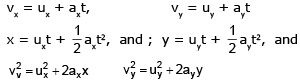where symbols have their usual meanings. Thus resultant motion would be described by the equations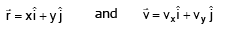4.1 PROJECTILE MOTION

It is the best example to understand motion in a plane. If we project a particle obliquely from the surface of earth, as shown in the figure below, then it can be considered as two perpendicular 1-D motions - one along the horizontal and other along the vertical.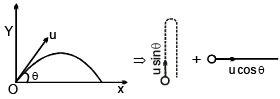Assume that effect of air friction and wind resistance are negligible and value of acceleration due to gravity  is constant.

Take point of projection as origin and horizontal and vertical direction as +ve X and Y-axes, respectively.
For X-axis For Y - axis
ux = u cosθ, uy = u sinθ
ax = 0, ay = – g,
vx = u cosθ, and vy = u sinθ – gt, and
x = u cosθ × t   y = u sinq t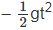It is clear from above equations that horizontal component of velocity of the particle remains constant while vertical component of velocity is first decreasing, gets zero at the highest point of trajectory and then increases in the opposite direction. At the highest point, speed of the particle is minimum.

The time, which projectile takes to come back to same (initial) level is called the time of flight (T).
At initial and final points, y = 0,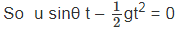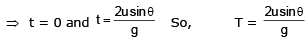Range (R) The horizontal distance covered by the projectile during its motion is said to be range of the projectile
R = u cosθ × T =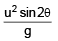For a given projection speed, the range would be maximum for q = 45°.
Maximum height attained by the projectile is
H =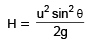at maximum height the vertical component of velocity is 0.
Time of ascent = Time of descent =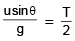• Speed, kinetic energy, momentum of the particle initially decreases in a projectile motion and attains a minimum value (not equal to zero) and then again increases.
• θ is the angle between  and horizontal which decreases to zero. (at top most point) and again increases in the negative direction.

Ex.33 A body is projected with a velocity of 30 ms–1 at an angle of 30° with the vertical. Find the maximum height, time of flight and the horizontal range.
Sol.
Here u = 30 ms–1,
Angle of projection, θ = 90 – 30 = 60°
Maximum height,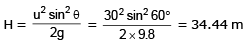Time of flight,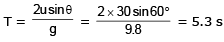Horizontal range,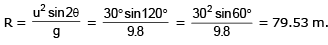Ex.34 Find out the relation between uA, uB, uC (where uA, uB, uC are the initial velocities of particles A, B, C, respectively)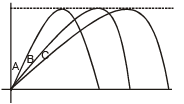Sol. ∵ Hmax is same for all three particle A, B, C
⇒⇒ uy is same for all       ∴  uyA = uyB = uyC
⇒ TA = TB = TC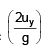from figure RC > RB > RA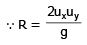⇒ uxC > uxB > uxA     ⇒ uA < uB< u

(C) Coordinate of a particle after a given time t :

Particle reach at a point P after time t then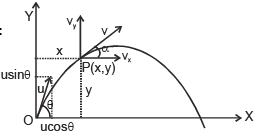x = ucosθ .t
y = usinθ.t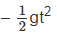Position vector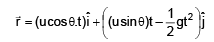(D) Velocity  and direction of motion after a given time :

After time 't' vx = ucosθ and vy = usinθ – gt
Hence resultant velocity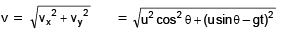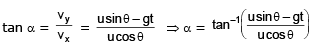(E) Velocity and direction of motion at a given height :

At a height 'h', vx = ucosθ
And vy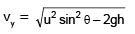∴ Resultant velocity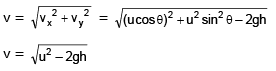Note that this is the velocity that a particle would have at height h if it is projected vertically from ground with u.

Ex.35 A body is projected with a velocity of 20 ms–1 in a direction making an angle of 60° with the horizontal. Calculate its (i) position after 0.5 s and (ii) velocity after 0.5 s.

Sol. Here u = 20 ms–1, q = 60° , t = 0.5 s

(i)  x = (u cos θ)t = (20 cos60°) × 0.5 = 5 m
y = (u sin θ) t= (20 × sin 60°) × 0.5
1/2 –  × 9.8 × (0.5)2 = 7.43 m
(ii) vx = u cos θ = 20 cos 60° = 10 ms–1
vy =  u sin θ – gt = 20 sin 60° – 9.8 × 0.5
= 12.42 ms–1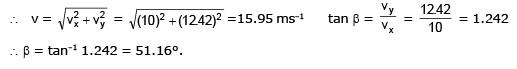Equation of trajectory of a projectile.

Suppose the body reaches the point P(x, y) after time t.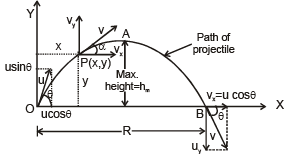∵ The horizontal distance covered by the body in time t,
x = Horizontal velocity × time = u cos θ. t
or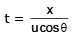For vertical motion : u = u sinθ, a = –g, so the vertical distance covered in time t is given by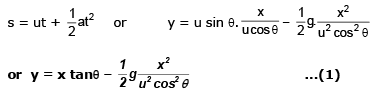or y = px – qx2, where p and q are constants.

Thus y is a quadratic function of x. Hence the trajectory of a projectile is a parabola.

From equation (1)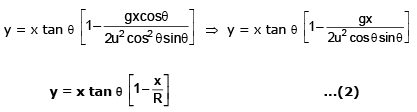Equation (2) is another form of trajectory equation of projectile

Ex.36 A ball is thrown from ground level so as to just clear a wall 4 m high at a distance of 4 m and falls at a distance of 14 m from the wall. Find the magnitude and direction of the velocity.
Sol.
The ball passes through the point P(4, 4). So its range = 4 + 14 = 18m.

The trajectory of the ball is,

Now x = 4m,  y = 4m and R = 18 m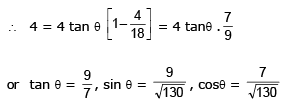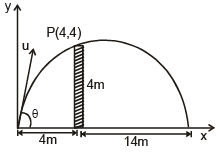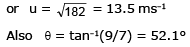Ex.37 A particle is projected over a triangle from one end of a horizontal base and grazing the vertex falls on the other end of the base. If a and b be the base angles and q the angle of projection, prove that tan q =  tan a + tan b.
Sol
. If R is the range of the particle, then from the figure we have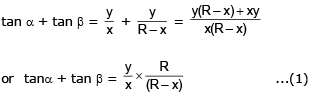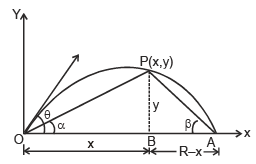Also, the trajectory of the particle is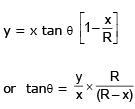From equations (1) and (2), we get
tan θ = tan α + tan β.

4.2 Projectile fired parallel to horizontal. As shown in shown figure suppose a body is projected horizontally with velocity u from a point O at a certain height h above the ground level. The body is under the influence of two simultaneous independent motions:

(i) Uniform horizontal velocity u.
(ii) Vertically downward accelerated motion with  constant acceleration g.
Under the combined effect of the above two motions, the body moves along the path OPA.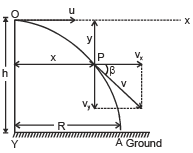Trajectory of the projectile. After the time t, suppose the body reaches the point P(x, y).
The horizontal distance covered by the body in time t is
x = ut ∴  t = x/u
The vertical distance travelled by the body in time t is given by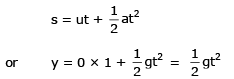[For vertical motion, u = 0]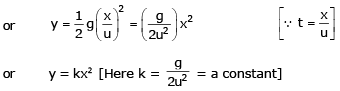As y is a quadratic function of x, so the trajectory of the projectile is a parabola.

Time of flight. It is the total time for which the projectile remains in its flight (from 0 to A). Let T be its time of flight.

For the vertical downward motion of the body,
we use
s = ut + 1/2 at2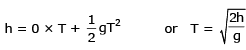The document Two-Dimensional Motion or Motion in a Plane - Notes | Study Physics Class 11 - NEET is a part of the NEET Course Physics Class 11.
All you need of NEET at this link: NEET

## Physics Class 11

127 videos|464 docs|210 tests

## Physics Class 11

127 videos|464 docs|210 tests

### How to Prepare for NEET

Read our guide to prepare for NEET which is created by Toppers & the best Teachers

Track your progress, build streaks, highlight & save important lessons and more!

,

,

,

,

,

,

,

,

,

,

,

,

,

,

,

,

,

,

,

,

,

;